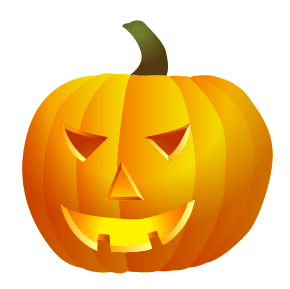# Halloween Pumpkin

Let’s have some fun for Halloween. Learn how to draw a realistic Halloween pumpkin in Illustrator. Perhaps you can attach to your eCard or email. This tutorial will focus on the Pen Tool, Pathfinder, and Gradient Tool.

Required: Illustrator 9+

### 1. Basic shapes

First, use the Pen tool and draw the basic shapes of the pumpkin.### 2. Fill Gradient

Fill the shapes with a linear gradient with no stroke fill. Gradient color1: R=255 G=127 B=0, color2: R=255 G=127 B=0Select all shapes, go to menu Edit > Copy (Ctrl+C). Then go to menu Edit > Paste in Front (Ctrl+F) to paste in position.With the shapes remain selected, open Pathfinder palette (Window > Pathfinder). Hold down Alt key, click on Add to shape area. This will merge shapes together.Select the following gradient setting – color1: R=255 G=255 B=255, color2: R=204 G=122 B=22. Slide the white picker toward the right side. With the merged shape remain selected, drag from the upper center toward bottom.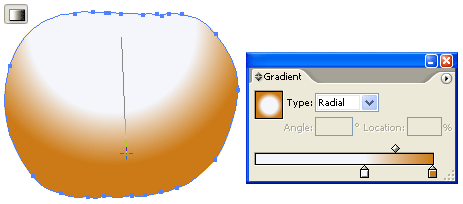Change the Blending Mode of the merged shape to Multiply.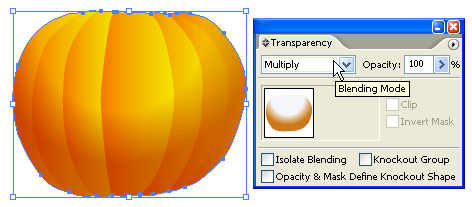### 4. Stem

Use the Pen tool and draw a shape of stem on top of the pumpkin. Fill it with a radial gradient as shown. Gradient color1: R=111 G=129 B=46, color2: R=82 G=56 B=17.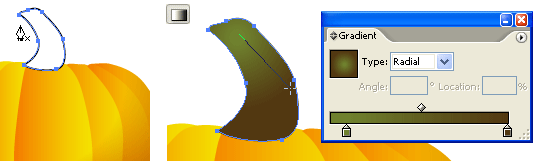### 5. Cutout Face

Make a new layer on top of the pumpkin. Use the Pen tool and draw the basic shapes of the face. Select all the shapes (face only), use the Gradient tool and fill it with a radial gradient as shown. Gradient color1: R=255 G=242 B=0, color2: R=255 G=146 B=0, color3: R=187 G=50 B=0.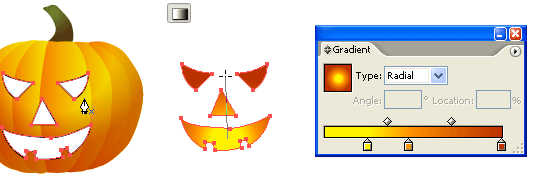### 6. Depth

Now, we need to add some depth to the pumpkin cutouts. Use the Pen tool, roughly draw a shape intersect with the left eye cutout. Then Copy the left eye and Paste in Front. Select the left eye shape and the intersect shape, go to Pathfinder, hold down Alt key and click on Intersect shape areas.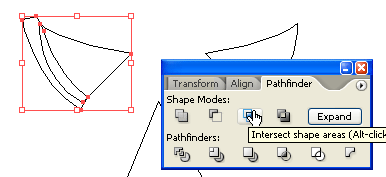Repeat this step until you get all the features done (eyes, nose, and mouth).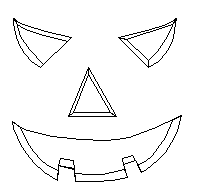### 7. Shadow & Highligh

Fill the shadow area with a darker gradient (color1: R=255 G=146 B=0, color2: R=187 G=50 B=0). Then fill the highlight area with a lighter gradient (color1: R=255 G=255 B=139, color2: R=255 G=164 B=0).### Final

That’s it!